# 数据分析项目：用户消费行为分析

• user_ud 用户ID
• order_dt: 购买日期
• order_products: 购买产品数
• order_amount: 购买金额

分析思路：
0：数据准备阶段（数据预处理）
1：按月数据分析
2：用户个体消费分析
3：用户消费行为分析4：复购率，回购率分析

0：数据准备

import pandas as pd
import numpy as np
import matplotlib.pyplot as plt
%matplotlib inline
import seaborn as sns
plt.rcParams['font.sans-serif']=['SimHei'] #用来正常显示中文标签

导入数据，设置相应字段

1 columns = ['user_id','order_dt','order_products','order_amount']
2 data = pd.read_table('CDNOW_master.txt',names=columns,sep='\s+')# 查看数据data.head()# 大概格式了解data.info（）# 数据字段  
• user_ud 用户ID
• order_dt: 购买日期
• order_products: 购买产品数
• order_amount: 购买金额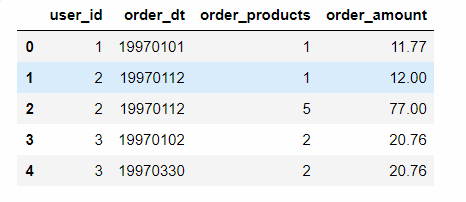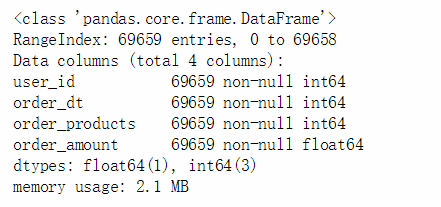data['order_dt'] = pd.to_datetime(data.order_dt,format='%Y%m%d')
# 新增一个月 （对月进行分析） values（数组形式） 因为很多是进行月份的一个处理
data['month'] = data.order_dt.values.astype('datetime64[M]')

data.info()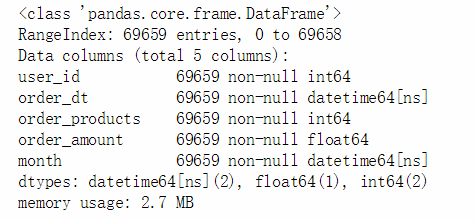# describe是描述统计data.describe()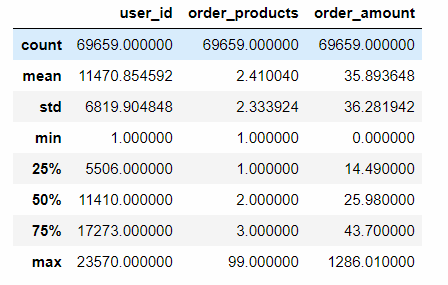• 观察数据，用户平均订单必输2.4个商品，标准差为2.3，略有波动，中位数书为2个商品，说明绝大部分订单的购买量不多，最大值为99，有一定的极值干扰
• 用户消费均值35元左右，中位数25元，最大金额达到了1286元，有一定极值干扰
　　1：按月数据分析
• 每月消费总金额
• 每月消费次数
• 每月产品购买量
• 每月的消费人数
# 按月分组
grouped_month = data.groupby('month')

fig = plt.figure(figsize = (8,12))
# 按月金额数
ax1.plot(grouped_month.order_amount.sum(),c='blue')
ax1.set_title('每月消费总金额变化趋势')
# 按月的订单数
ax2.plot(grouped_month.user_id.count(),c='blue')
ax2.set_title('每月订单数变化趋势')
# 按月产品数
ax3.plot(grouped_month.order_products.sum(),c='blue')
ax3.set_title('每月消费产品购买量')
# 每月用户人数  这里需要去重，有些用户重复购买
ax4.plot(grouped_month.user_id.apply(lambda x:len(x.drop_duplicates())),c='blue')
ax4.set_title('每月用户数变化趋势')
按月进行统计，下面通过折线图分别查看数据变化趋势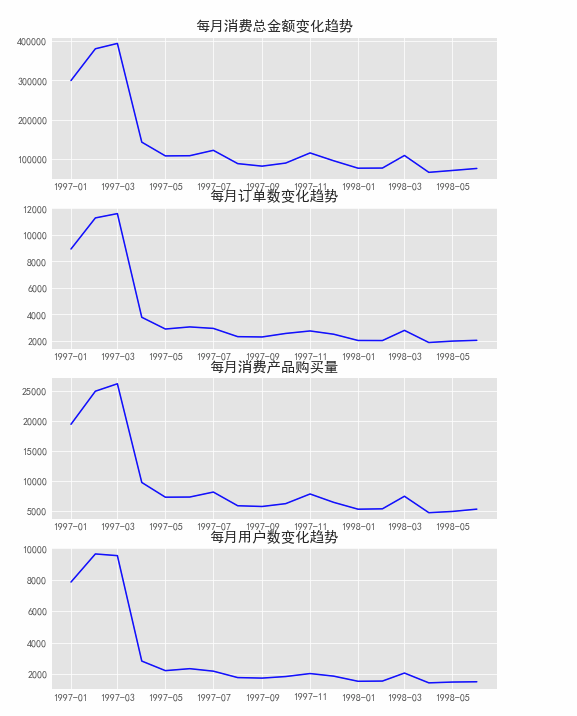data.pivot_table(index='month',
values = ['order_products','order_amount','user_id'],
aggfunc = {'order_products':'sum',
'order_amount':'sum',
'user_id':'count'})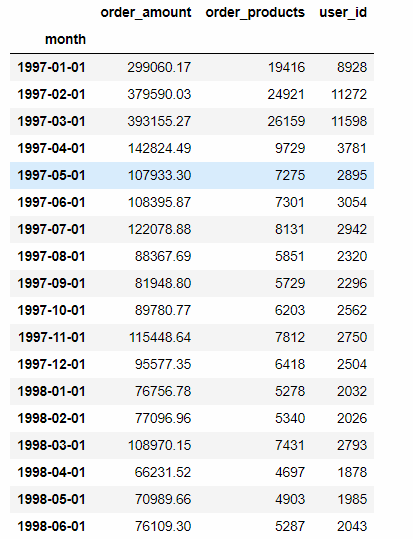## 2.用户个体消费分析

• 用户消费金额，消费次数描述统计
• 用户消费金额和消费次数的散点图
• 用户消费金额的分布图
• 用户消费次数的分布图
• 用户累积消费金额占比（百分之多少的用户占了百分之多少的消费额）

01-用户消费金额和消费次数的描述统计grouped_user = data.groupby('user_id')
grouped_user.sum().describe()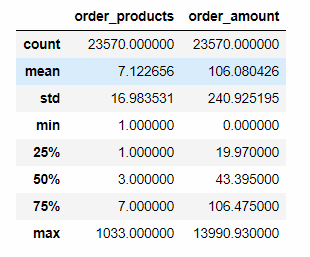• 用户平均购买量7张CD,标准差17，波动比较大，但是中位值只有3，说明小部分用户购买了大量的CD
• 用户平均消费为106元，中位值为43，也有极值干扰
• 消费的话一般都会符合二八法则

02 - 用户消费金额和消费次数散点图# 设置排除一些极值的干扰进行观察grouped_user.sum().query('order_amount < 4000').plot.scatter(x='order_amount',y='order_products')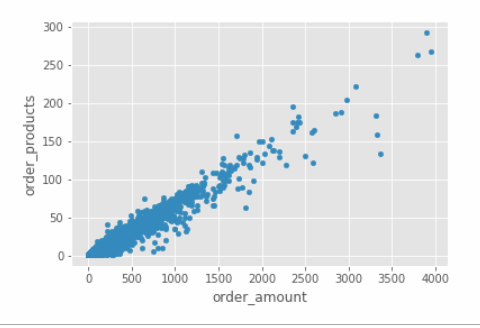• 绘制散点图，由于产品比较单一，购买金额和产品数呈线性关系
03-用户消费金额的分布图（二八法则）grouped_user.sum().order_amount.plot.hist(bins=20)# bins=20，就是分成20块，最高金额是14000，每个项就是700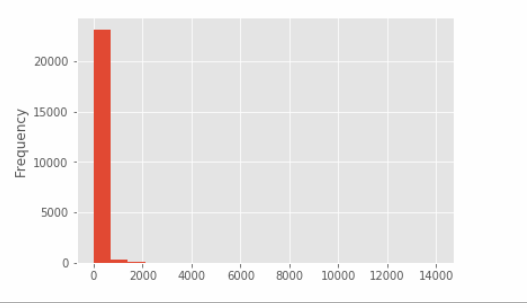• 由直方图可知，用户消费金额，大部分消费金额能力确实不高，绝大部分呈几种在很低的消费档次，高消费用户在图中基本看不到，这也符合消费行为的行业规律
• 小部分异常值干扰判断，可以过滤操作排除异常
04-用户消费次数分布图grouped_user.sum().query('order_products<92').order_products.hist(bins = 40)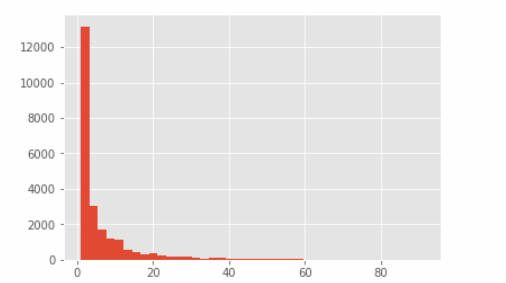• 使用切比雪夫定理过滤异常值，计算95%的数据分布情况  （均值+5*标准差）
05 -用户累计消费金额占比user_cumsum = grouped_user.sum().sort_values('order_amount').apply(lambda x: x.cumsum() / x.sum())
user_cumsum.reset_index().order_amount.plot()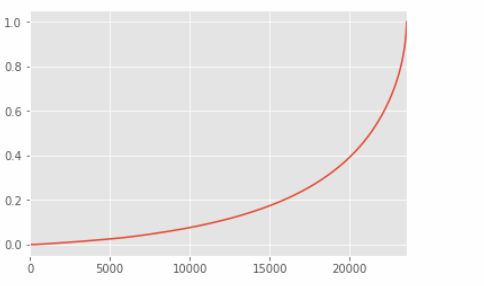• 通过观察，50%的用户仅仅贡献了15%的消费额度，而排名前五千用户就贡献了%60的消费额
print(user_cumsum.head(8))
print(user_cumsum.tail(8))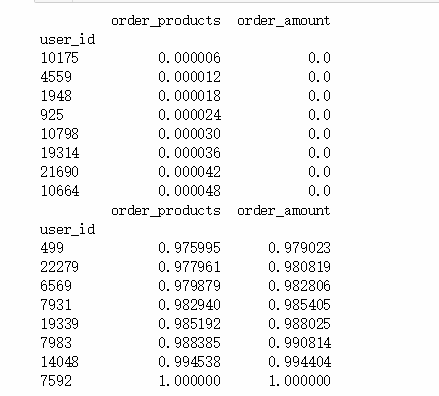• 上面是一些累积占比的数据，数据中存在金额为0，可能是一些活动赠送等原因

## 3.用户消费行为分析

• 用户第一次消费（首购）
• 用户最后一次消费
• 新老客户消费比
• 多少用户仅仅消费了一次
• 每月新客占比
• 用户分层
• RFM
• 新、老、活跃、回流、流失/不活跃
• 用户购买周期（按订单）
• 用户消费周期描述
• 用户消费周期分布
• 用户生命周期（按第一次 & 最后一次消费）
• 用户生命周期描述
• 用户生命周期分布
01-第一次购买时间分布# min()  时间最小，第一次购买
grouped_user.min().order_dt.value_counts().plot()
# 2月发生较大下跌  渠道发生变化，或者其他  可以做一些假设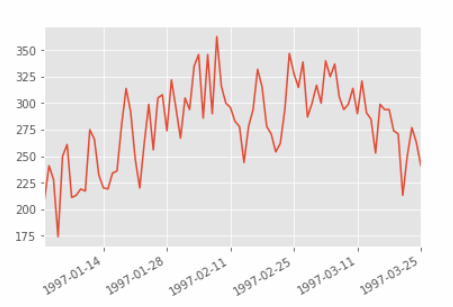• 用户第一次购买分布，集中在前三个月，其中2月11日至2月25日有一次剧烈波动
02-最后一次购买时间分布grouped_user.max().order_dt.value_counts().plot()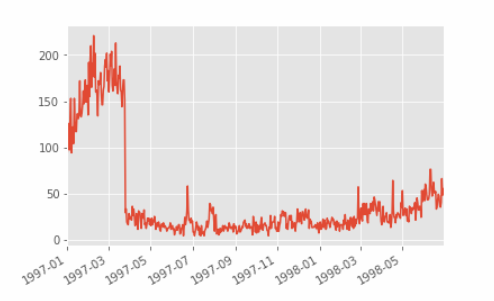• 用户最后一次购买的分布比第一次购买分布广
• 大部分最后一次购买在前三个月，说明很多用户购买一次后就不再进行购买
• 随着时间递增，最后一次购买数在递增，消费呈线性流失上升的情况
03-用户第一次购买与最后一次购买 新老客的消费比user_life =grouped_user.order_dt.agg(['min','max'])
user_life.head()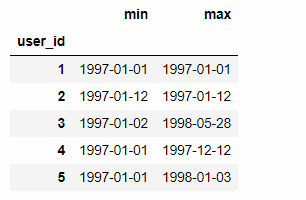(user_life['min'] == user_life['max']).value_counts()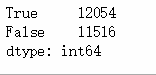rate = (user_life['min'] == user_life['max']).value_counts()
labels = ['只消费一次用户','多次消费用户']
plt.axis('equal') # 保证长宽相等
plt.pie(rate,explode=(0,0.15),labels=labels,autopct='%2.1f%%',startangle=90,colors=['r','orange'],radius=1.5)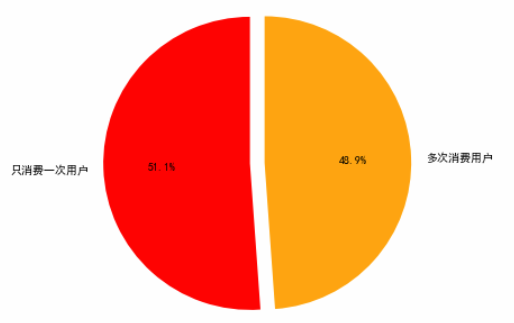True 第一次购买时间和最后一次购买时间一样，有一般用户只消费了一次

04-用户分层
#  RFM   消费额 次数  最近一次消费 进行透视
rfm = data.pivot_table(index = 'user_id',
values = ['order_products','order_amount','order_dt'],
aggfunc = {'order_dt':'max',
'order_amount':'sum',
'order_products':'sum'})
rfm.head()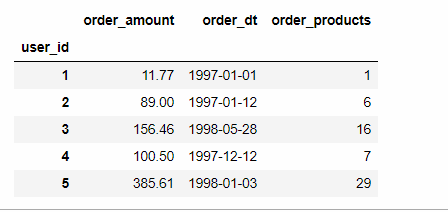# 表示离最近一次消费的时间间隔
-(rfm.order_dt - rfm.order_dt.max()).head()# 数值越大，离现在的时间越长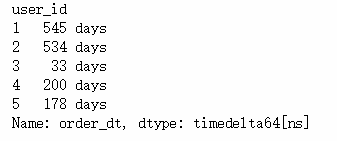# /np.timedelta64(1,'D')  消除单位   进行重命名
rfm['R'] = -(rfm.order_dt - rfm.order_dt.max()) / np.timedelta64(1,'D')
rfm.rename(columns = {'order_products':'F','order_amount':'M'},inplace=True)
rfm.head()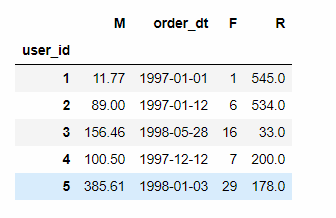rfm[['R','F','M']].apply(lambda x:x-x.mean()).head()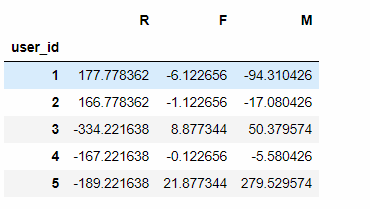R：离最近一次购买的天数   F：产品数（消费次数） M：消费金额   与均值的对比

def rfm_func(x):
level = x.apply(lambda x:'1' if x>= 0 else '0')　　# 字符串拼接　　# 111，R>0,是距离平均消费时间要久，R越大 说明没有消费时间越久  ，F >0 M>0,消费次数和金额也是较高的，重要价值客户，依次类推
label = level.R + level.F + level.M
d = {
'111':'重要价值客户',
'011':'重要保持客户',
'101':'重要挽留客户',
'001':'重要发展客户',
'110':'一般价值客户',
'010':'一般保持客户',
'100':'一般挽留客户',
'000':'一般发展客户'
}
result = d[label]
return result
# x - x.mean() （具体真实情况可以修改，不一定需要用均值）   切比雪夫也可以 > 200 极值人工处理掉
rfm['label'] = rfm[['R','F','M']].apply(lambda x:x-x.mean()).apply(rfm_func,axis=1)rfm.head()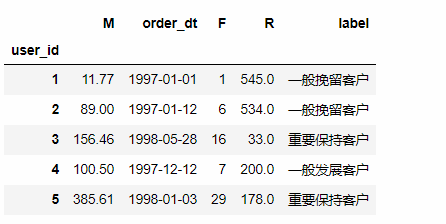rfm.groupby('label').sum()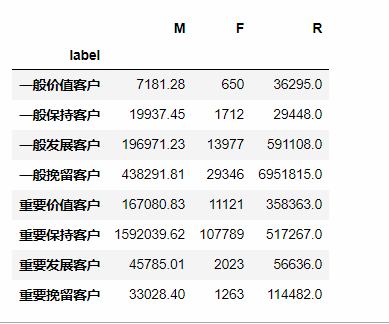rfm.groupby('label').count()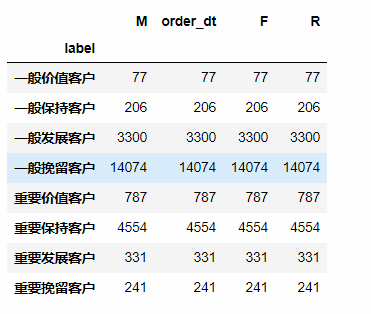# 各类类型用户占比use_c = rfm.groupby('label').count()
plt.axis('equal')
labels = ['一般价值客户','一般保持客户','一般发展客户','一般挽留客户','重要价值客户','重要保持客户','重要发展客户','重要挽留客户']
plt.pie(use_c['M'],
autopct='%3.1f%%',
labels = labels,
pctdistance=0.9,
labeldistance = 1.2,
startangle = 15)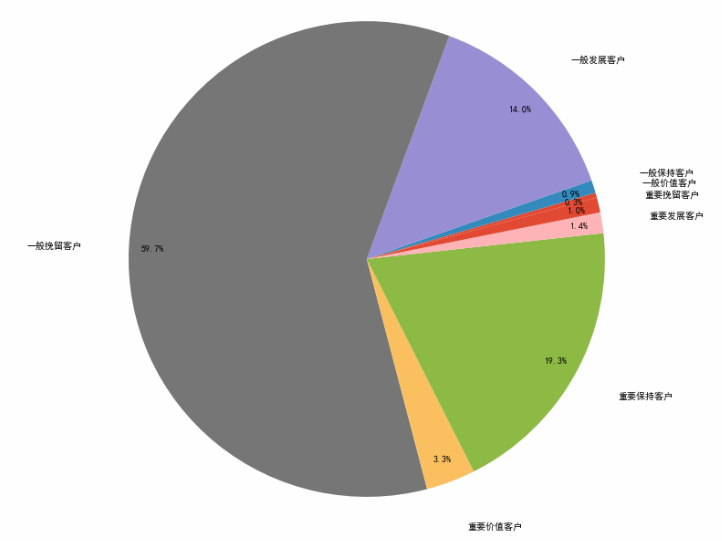# 对应标签，使用不同颜色表示# 绿色为重要价值客户，红色为非重要价值用户rfm.loc[rfm.label == '重要价值客户','color'] = 'g'
rfm.loc[~(rfm.label == '重要价值客户'),'color'] = 'r'
rfm.plot.scatter('F','R',c=rfm.color)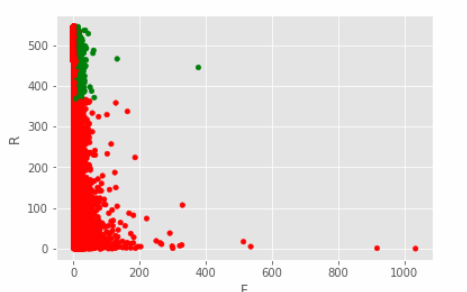rfm.head()
# rfm 象限法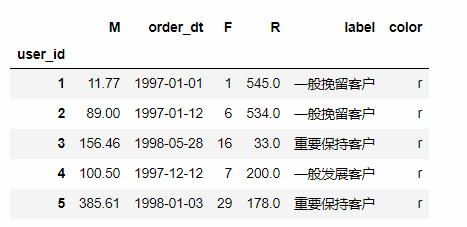rfm.groupby('label').sum()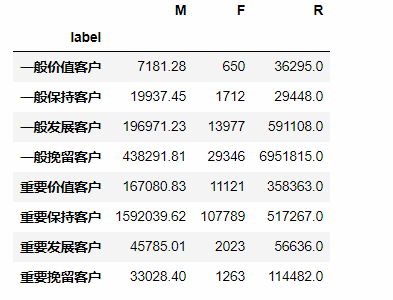• 尽量用小部分的用户覆盖大部分的额度
• 不要为了数据好看划分等级
• 极值会拉均值
• 根据数据可以和业务相结合，如何提升一些重要的指标
06-用户生命周期pivoted_counts = data.pivot_table(index = 'user_id',
columns = 'month',
values = 'order_dt',
aggfunc = 'count').fillna(0)
# 按月份进行对比，1月份哪些是购买的，再去对比二月份哪些是购买的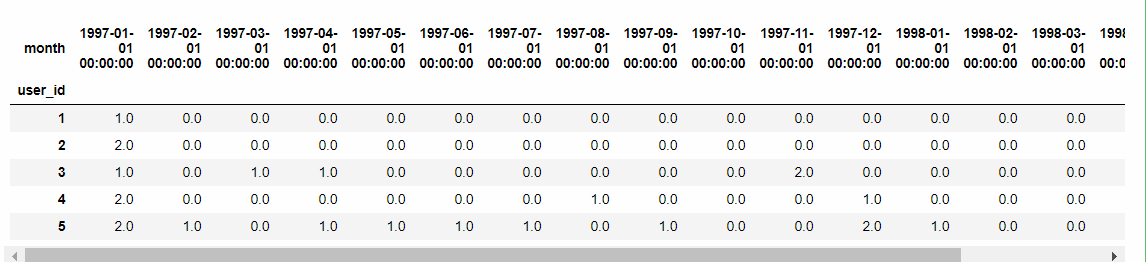消费过的为1 ，没消费过的为0data_purchase = pivoted_counts.applymap(lambda x: 1 if x > 0 else 0)
data_purchase.tail()
# 透视会补上  第一次从3月分开始购买 前面补成0 ， 需要进行判，第一次消费作为生命周期的起始
# se.columns.values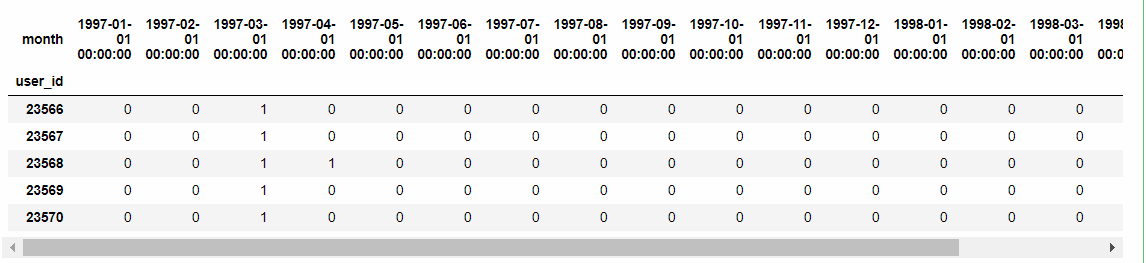def active_status(data):
status = []
for i in range(18):

#若本月没有消费
if data[i] == 0:
if len(status) > 0:
if status[i-1] == 'unreg':
status.append('unreg')
else:
status.append('unactive')
else:
status.append('unreg')
#若本月消费
else:
if len(status) == 0:
status.append('new')
else:
if status[i-1] == 'unactive':
status.append('return')
elif status[i-1] == 'unreg':
status.append('new')
else:
status.append('active')　　# 这里需要对返回的值进行转换，将列表转为Series
return pd.Series(status, index = pivoted_counts.columns)
• 若本月没有消费
• 若之前是未注册，则依旧未注册
• 若之前有消费，则为流失/不活跃
• 其他情况，为未注册
• 若本月有消费
• 若是第一次消费，则为新用户
• 如果之前有过消费，则上个月为不活跃，则为回流
• 如果上个月为未注册，则为新用户
• 除此之外，为活跃
purchase_stats = data_purchase.apply(active_status,axis=1,result_type ='expand')
# df.rename(columns={ df.columns: "new name" }, inplace=True)
# purchase_stats.rename(columns={purchase_stats.columns:data_purchase.columns})
purchase_stats.head()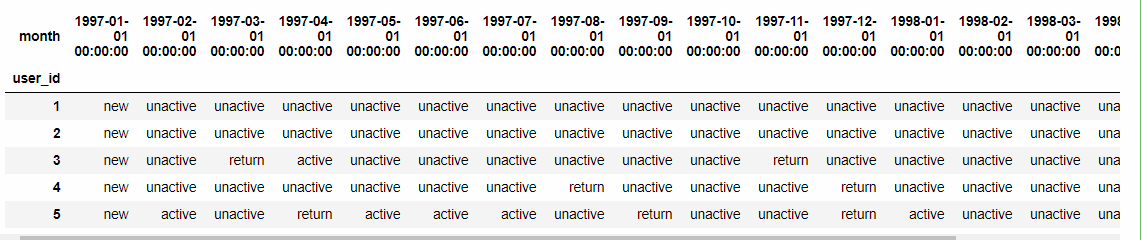purchase_status_ct = purchase_stats.replace('unreg',np.NaN).apply(lambda x : pd.value_counts(x))
purchase_status_ct
# 未注册不希望参与处理  设置我空值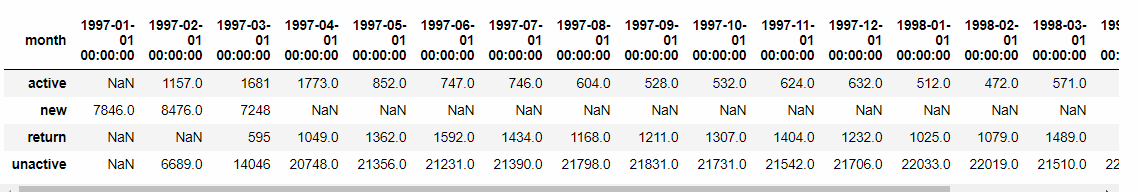新用户转换为是否活跃等等 流失用户在增加

# 各个状态的占比
purchase_status_ct.fillna(0).T.apply(lambda x:x/x.sum(),axis=1)
purchase_status_ct.fillna(0).T.plot.area()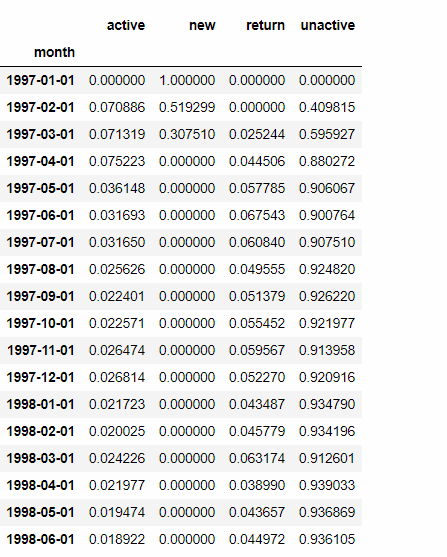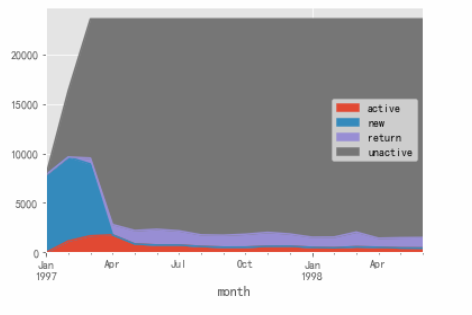• 活跃用户，持续消费的用户，对应的使消费运营质量
• 回流用户，之前不消费本月才消费，对应的使唤回运营
• 不活跃用户，对应的是流失
用户购买周期 data.order_dt.head(10)
# 把所有的数据进行一个错位  DataFrame.shift data.order_dt.shift().head(10)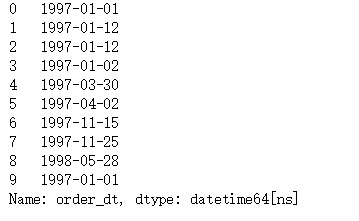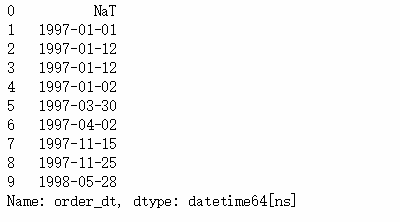#   一个用户可能多个订单  每个订单的间隔
order_diff = grouped_user.apply(lambda x:x.order_dt - x.order_dt.shift())
order_diff.head(10)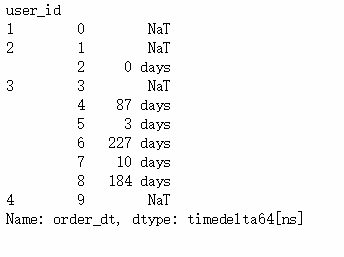ID为3的用户          3-4间隔了87天

NaT表示一个订单

order_diff.describe()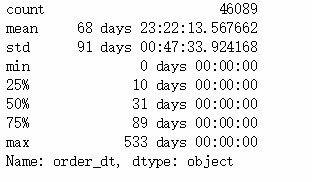# 去除单位  间隔天数分布图(order_diff / np.timedelta64(1,'D')).hist(bins=20) order_diff / np.timedelta64(1,'D')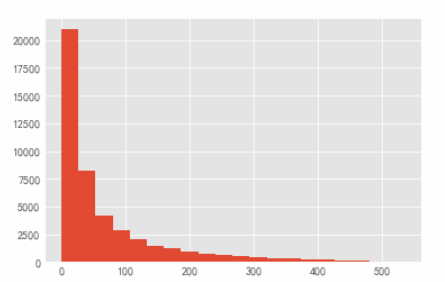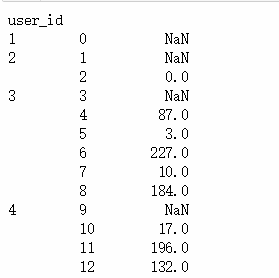(user_life['max'] - user_life['min']).describe()
# 整体的生命周期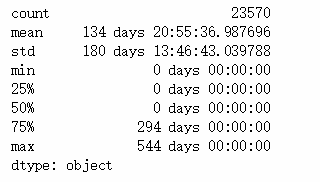((user_life['max'] - user_life['min']) / np.timedelta64(1,'D')).hist(bins = 40)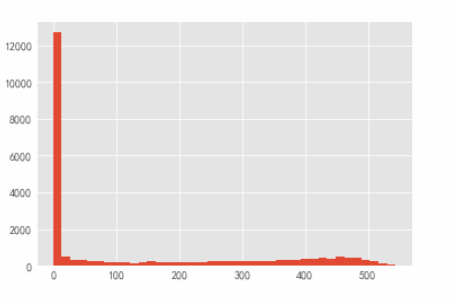• 用户的生命周期受只购买一次的用户影响比较厉害 （可以进行排除 当特殊情况，想提取出购买一次以上的用户）
• 用户均消费134天，中位数仅为0
u_l = ((user_life['max'] - user_life['min']).reset_index() / np.timedelta64(1,'D'))
u_l[u_l > 0].hist(bins=40)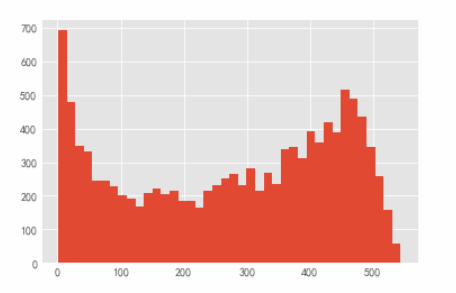• 左边比较高，还是有些用户的生命周期是比较短的，例如10、20天等
• 有不少用户生命周期也是比较稳定

## 4.复购率和回购率分析

• 复购率
• 自然月内，购买多次的用户占比
• 回购率
• 曾今购买过的用户在某一时期内的再次购买占比
pivoted_counts.head()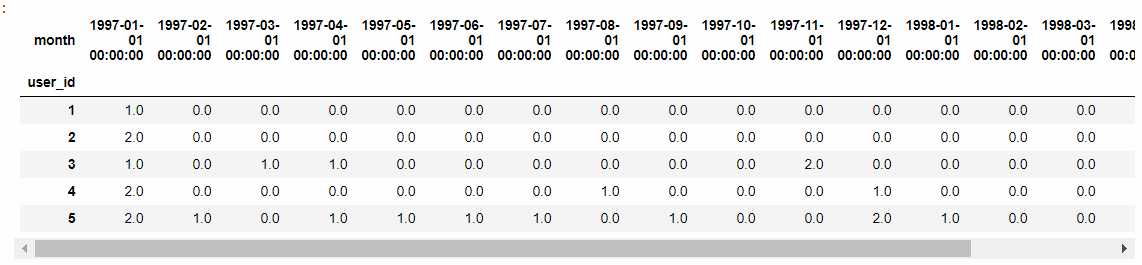# 购买大于1次的 赋值为1 ，然后小于等于1 的 如果是购买次数是0，则赋值为空，否则 就是购买一次，赋值为0purchase_r = pivoted_counts.applymap(lambda x: 1 if x > 1 else np.NaN if x == 0 else 0)
purchase_r.head()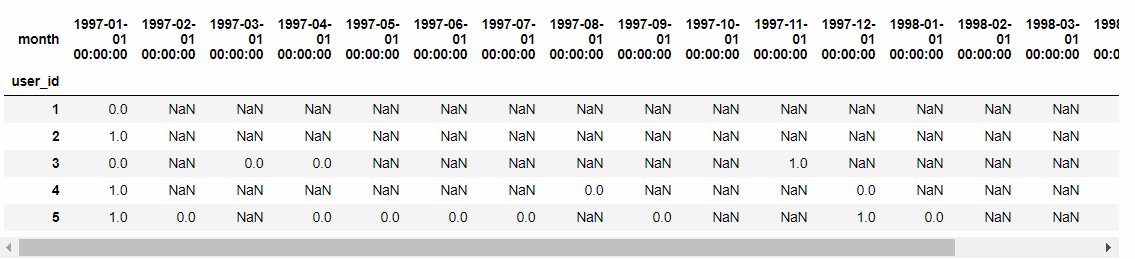# sum（） 0 不计数，count（） 0计数(purchase_r.sum() / purchase_r.count()).plot(figsize = (10,4))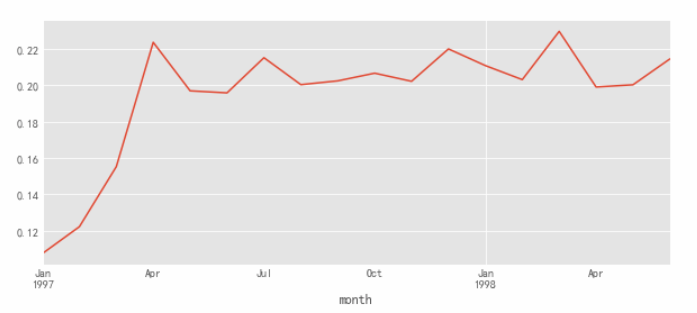• 复购率稳定在20% 左右，前三个月因为有大量新用户涌入，而这些用户只购买了一次，所以导致复购率较低
回购率 只需要0  1 代表data_purchase.head()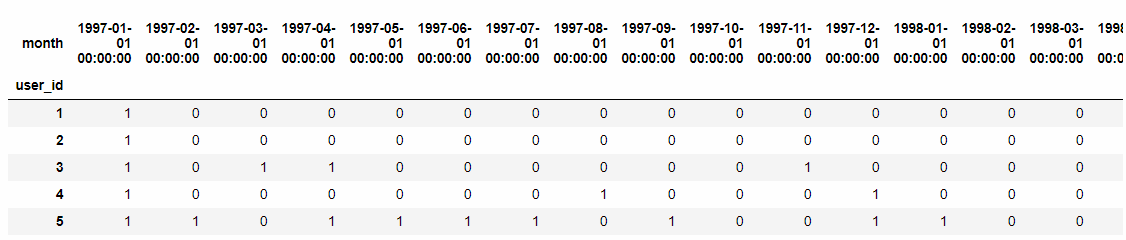def purchase_back(data):
status = []
for i in range(17):
if data[i] == 1: # 本月进行过消费
if data[i+1] == 1: # 下一月是否进行消费
status.append(1) #消费为1 回购了
if data[i+1] == 0:
status.append(0) # 未消费则为0 没有回购
else:
status.append(np.NaN) # 之前没消费则不计
status.append(np.NaN) # 最后一个月没有判断需要补上
return pd.Series(status,data_purchase.columns)
purchase_b = data_purchase.apply(purchase_back, axis =1)
purchase_b.head(5)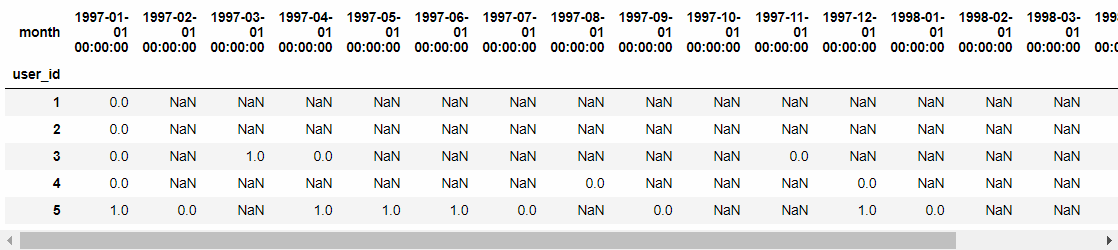• 0 表示上个月购买了，下个月没有进行消费，则是没有回购 ，
• 1代表当月消费过次月依旧消费，表示回购了
• NAN表示当月没有消费（不进行计算）
sum() 0 1进行求和，表示次月消费过的(purchase_b.sum() / purchase_b.count()).plot(figsize = (10, 4))•  回购率在30%左右波动，比复购率要高，综合分析得出，新客整体质量低于老客，老客忠诚度（回购率）表现较好，消费频次稍次，这个是是CDNow网站的用户消费特征

posted @ 2019-04-26 15:53  东西  Views(2391)  Comments(0Edit  收藏